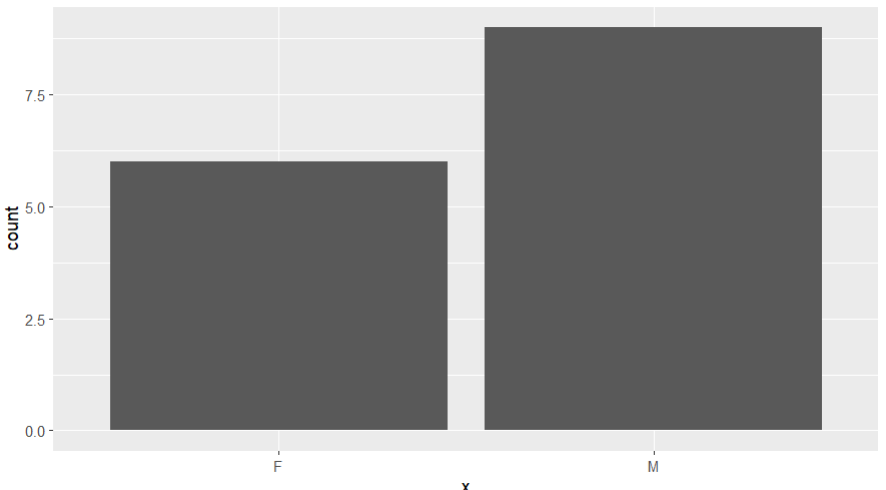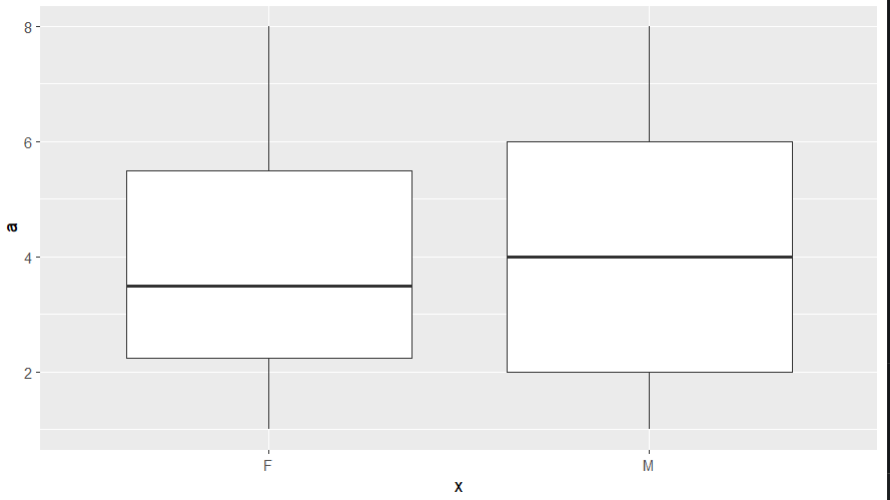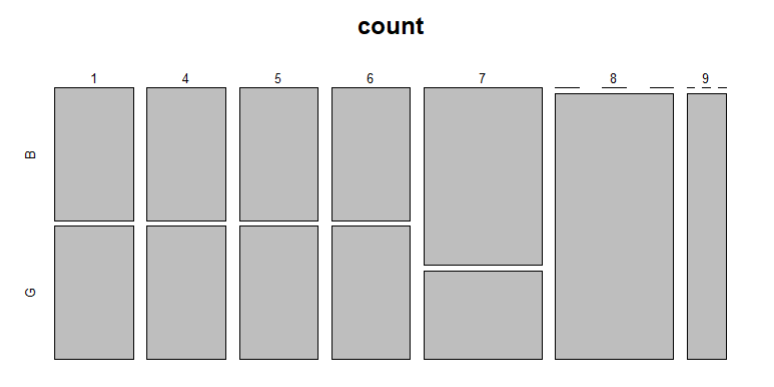# How to Plot Categorical Data in R?

• Last Updated : 19 Dec, 2021

In this article, we will be looking at different plots for the categorical data in the R programming language.

Categorical Data is a variable that can take on one of a limited, and usually fixed, a number of possible values, assigning each individual or other unit of observation to a particular group or nominal category on the basis of some qualitative property.

## Method 1: Create a bar plot of the categorical data

In this approach to create a bar plot of the categorical data, the user has to first install and import the ggplot2 package in the working R console, here the ggplot2 package is responsible to plot the ggplot2 barplot and providing various functionalities, then the user needs to call the geom_bar() function with the categorical data and then this will be returning a ggplot2 bar plot to the user containing the provided categories and their corresponding frequencies in the R programming language.

geom_bar() function is used to make the height of the bar proportional to the number of cases in each group.

Syntax:

`geom_bar()`

Example: Plotting bar graph of categorical data

## R

 `library``(ggplot2) ` ` `  `data < - ``data.frame``(x=``c``(``'M'``, ``'F'``, ``'M'``, ``'F'``, ``'M'``, ``'F'``, ` `                        ``'M'``, ``'F'``, ``'M'``, ``'F'``, ``'M'``, ``'F'``, ` `                        ``'M'``, ``'M'``, ``'M'``), ` `                    ``y=``c``(``'B'``, ``'G'``, ``'B'``, ``'B'``, ``'G'``, ``'G'``, ``'B'``, ` `                        ``'G'``, ``'G'``, ``'B'``, ``'G'``, ``'G'``, ``'B'``, ``'G'``, ` `                        ``'G'``), ` `                    ``a=``c``(8, 6, 6, 1, 2, 3, 7, 4, 4, 2, 5, ` `                        ``8, 1, 3, 2), ` `                    ``b=``c``(5, 7, 7, 4, 5, 6, 7, 8, 8, 6, 9, ` `                        ``4, 1, 8, 1)) ` ` `  `ggplot``(data, ``aes``(x=x)) + ``geom_bar``() `

Output:## Method 2: Create Boxplots by Group of categorical data

In this method to create the boxplot by a group of the given categorical data, the user needs to install and import the ggplot2 package to provide its functionalities and then the user simply needs to call the geom_box() function with the given data to plot a ggplot2 boxplot by the group in the R programming language.

Syntax:

`geom_box()`

Example: Plotting boxplot of categorical data

## R

 `library``(ggplot2) ` ` `  `data < - ``data.frame``(x=``c``(``'M'``, ``'F'``, ``'M'``, ``'F'``, ``'M'``, ``'F'``, ``'M'``, ``'F'``, ` `                        ``'M'``, ``'F'``, ``'M'``, ``'F'``, ``'M'``, ``'M'``, ``'M'``), ` `                    ``y=``c``(``'B'``, ``'G'``, ``'B'``, ``'B'``, ``'G'``, ``'G'``, ``'B'``, ``'G'``, ` `                        ``'G'``, ``'B'``, ``'G'``, ``'G'``, ``'B'``, ``'G'``, ``'G'``), ` `                    ``a=``c``(8, 6, 6, 1, 2, 3, 7, 4, 4, 2, 5, 8, 1, ` `                        ``3, 2), ` `                    ``b=``c``(5, 7, 7, 4, 5, 6, 7, 8, 8, 6, 9, 4, 1, ` `                        ``8, 1)) ` ` `  `ggplot``(data, ``aes``(x, y=a)) + ``geom_boxplot``() `

Output:## Method 3: Create Mosaic Plot of categorical data

In this method, the user has to simply call the mosaicplot() function with the data passed into this function as the parameter to get the resulting plot as the mosaic plot of the given categorical data in the R language.

mosaicplot() function is used to plot a mosaic on the current graphics device.

Syntax:

mosaicplot(x,)

Parameters:

• x: a contingency table in array form, with optional category labels specified

Example: Plotting mosaic plot of categorical data

## R

 `data < - ``data.frame``(x=``c``(``'M'``, ``'F'``, ``'M'``, ``'F'``, ``'M'``, ``'F'``, ``'M'``, ``'F'``, ` `                        ``'M'``, ``'F'``, ``'M'``, ``'F'``, ``'M'``, ``'M'``, ``'M'``), ` `                    ``y=``c``(``'B'``, ``'G'``, ``'B'``, ``'B'``, ``'G'``, ``'G'``, ``'B'``, ``'G'``, ` `                        ``'G'``, ``'B'``, ``'G'``, ``'G'``, ``'B'``, ``'G'``, ``'G'``), ` `                    ``a=``c``(8, 6, 6, 1, 2, 3, 7, 4, 4, 2, 5, 8, 1, ` `                        ``3, 2), ` `                    ``b=``c``(5, 7, 7, 4, 5, 6, 7, 8, 8, 6, 9, 4, 1, ` `                        ``8, 1)) ` ` `  `count < - ``table``(data\$b, data\$y) ` ` `  `mosaicplot``(count) `

Output:My Personal Notes arrow_drop_up
Recommended Articles
Page :